Latest Banking jobs   »   Quantitative Aptitude Quiz For IBPS Clerk/NIACL...

# Quantitative Aptitude Quiz For IBPS Clerk/NIACL AO Prelims 2021- 3rd September

Directions (1-5): Solve the following equations and mark the correct option given below.
(a) if x>y
(b) if x≥y
(c) if y>x
(d) if y≥x
(e) if x=y or no relation can be established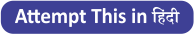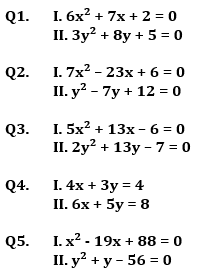Directions (6-10): In each of the following questions, two equations (I) and (II) are given you have to solve both the equations and give answer.
(a) If x > y
(b) If x ≥ y
(c) If x < y
(d) If x ≤ y
(e) If x = y or no relation can be established between x and y.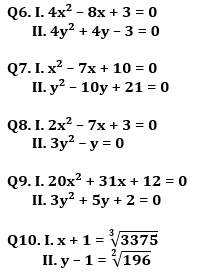Directions (11-15): In the following questions, two equations (I) and (II) are given. You have to solve both the equations and mark the appropriate answer.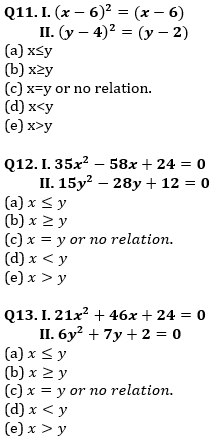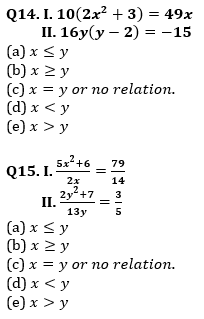Solutions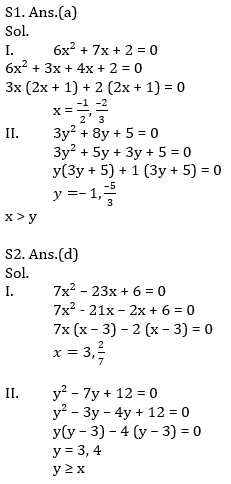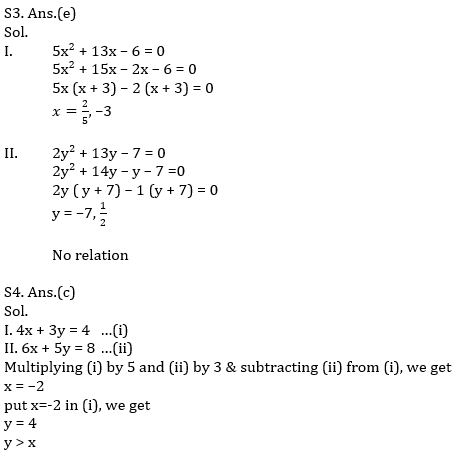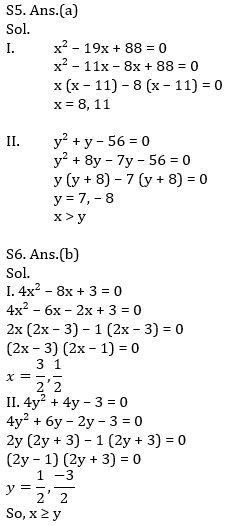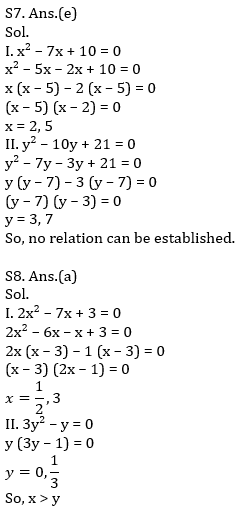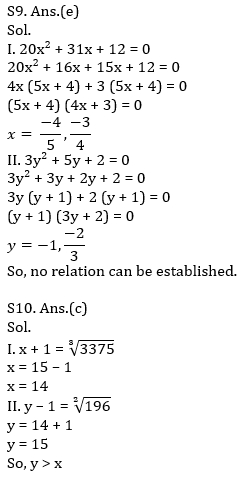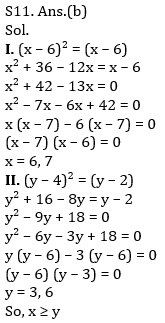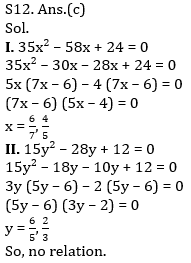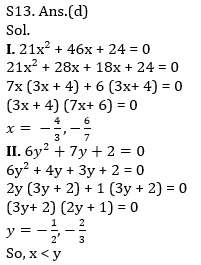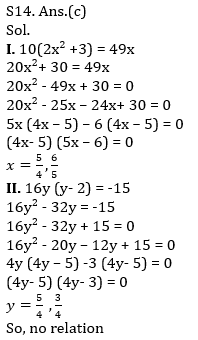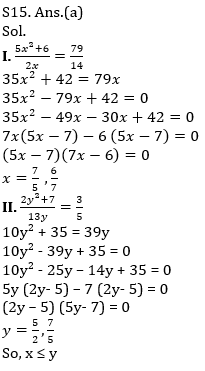Click Here to Register for Bank Exams 2021 Preparation Material#### Congratulations!Download Hindu Review of October 2021: Free PDF